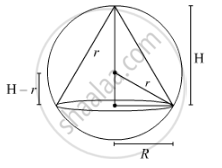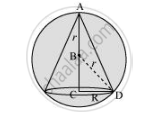# Show that the Altitude of the Right Circular Cone of Maximum Volume that Can Be Inscribed in a Sphere of Radius R Is (4r)/3. - Mathematics and Statistics

Show that the altitude of the right circular cone of maximum volume that can be inscribed in a sphere of radius r is (4r)/3. Also find maximum volume in terms of volume of the sphere

Show that the altitude of a right circular cone of maximum volume that can be inscribed in a sphere of radius r is (4r)/3 . Also, show that the maximum volume of the cone is 8/27  of the volume of the sphere.

#### Solution 1V=1/3piR^2H
It is clear from the figure that

R^2+(H−r)^2=r^2

⇒R^2+H^2+r^2−2Hr=r^2

⇒R^2=2Hr−H^2

Substituting the value of R2 in the formula for the volume of the cone, we get

V=1/3pi(2Hr-H^2)H

 V=2/3πrH^2−π/3H^3

Differentiating with respect to H both sides, we get:

(dV)/(dH)=4/3πrH−πH^2

At critical point,(dV)/(dH) is 0.

⇒4/3πrH−πH^2=0

⇒H=4/3r

Differentiating V w.r.t H again, we get:

(d^2V)/(dH^2)=4/3πr−2πH

|(d^2V)/(dH^2)|_(H=4/3r)=−4/3πr <0

Hence maxima.

Volume of cone =
1/3πR^2H

 V=2/3πrH^2−π/3H^3

Substituting the value of H, we get:

V=2/3πr(4/3r)^2−π/3(4/3r)^3

=>V=8/27(4πr^3−8/3πr^3)

=>V=8/27(4/3πr^3)

=>V=8/27(volume of sphere)

#### Solution 2

A sphere of fixed radius (r) is given.

Let R and h be the radius and the height of the cone, respectively.The volume (V) of the cone is given by,

V=1/3piR^2h

Now, from the right triangle BCD, we have:

BC=sqrt(r^2-R^2)

:.h=r+sqrt(r^2-R^2)

V=1/3piR^2(r+sqrt(r^2-R^2))=1/3piR^2r+1/3piR^2sqrt(r^2-R^2)

(dV)/(dR)=2/3piRr+2/3piRsqrt(r^2-R^2)+(piR^2)/3 (-2R)/(2sqrt(r^2-R^2))

=2/3piRr+2/3piRsqrt(r^2-R^2)-(piR^3)/(3sqrt(r^2-R^2))

=2/3piRr+(2piR(r^2-R^2)-piR^3)/(3sqrt(r^2-R^2))

2/3piRr+(2piRr^2-3piR^3)/(3sqrt(r^2-R^2))

Now

(dV)/(dR^2)=0

=>(2pirR)/3=(3piR^3-2piRr^2)/(3sqrt(r^2-R^2))

=>2rsqrt(r^2-R^2)=3R^2-2r^2

=>4r^2(r^2-R^2)=(3R^2-2r^2)^2

=>4r^4-4r^2R^2=9R^4+4r^4-12R^2r^2

=>9R^4-8r^2R^2=0

=>9R^2=8r^2

=>R^2=(8r^2)/9

Now,

(d^2V)/(dR^2)=(2pir)/3+(3sqrt(r^2-R^2)(2pir^2-9piR^2)-(2piRr^2-3piR^3)(-6R)1/(2sqrtr^2-R^2))/(9(r^2-R^2))

=(2pir)/3+(3sqrt(r^2-R^2) (2pir^2-9piR^2)+(2piRr^2-3piR^3)(3R)1/(2sqrt(r^2-R^2)))/(9(r^2-R^2))

Now when R^2=(8r^2)/9it can be shown that (d^2V)/(dR^2)<0

∴ The volume is the maximum when R^2=(8r^2)/9

When R^2=(8r^2)/9height of cone= r+sqrt(r^2-(8r^2)/9)=r+sqrt(r^2/9)=r+r/3=(4r)/3

Hence, it can be seen that the altitude of a right circular cone of maximum volume that can be inscribed in a sphere of radius r is (4r)/3

Let volume of the sphere be V_s=4/3pir^3

r=3sqrt((3V_s)/(4pi))

Volume of cone, V = 1/3piR^2h

R (2sqrt2)/3r

V = 1/3pi((2sqrt2)/3r)xx(4r)/3

V 1/3pi(8r^2)/9xx(4r)/3

V=(32pir^3)/81=32/81pi[(3V_s)/(4pi)]

Volume of cone in terms of sphere

Concept: Maxima and Minima
Is there an error in this question or solution?